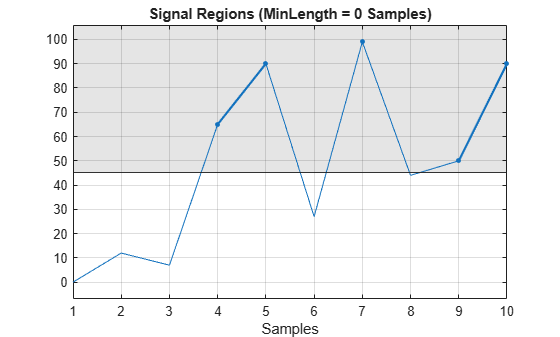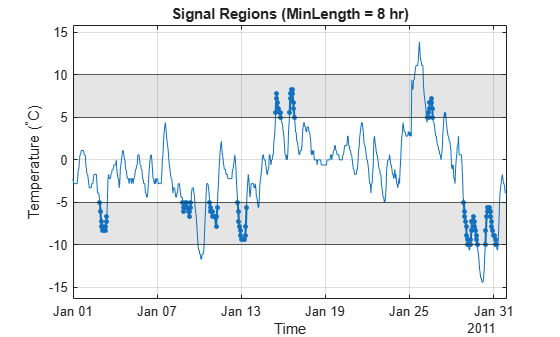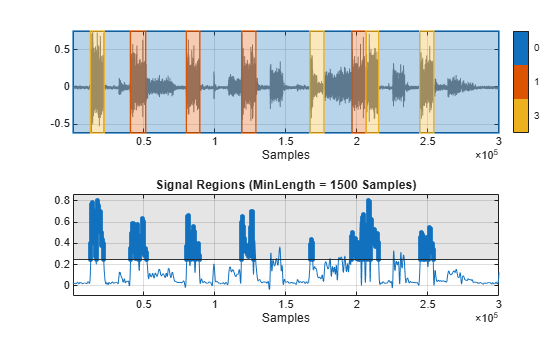Label signal samples with values within a specified range

Since R2023a

## Syntax

``msk = sigrangebinmask(x,bound)``
``msk = sigrangebinmask(x,bound,fs)``
``msk = sigrangebinmask(x,bound,ts)``
``msk = sigrangebinmask(___,Name=Value)``
``sigrangebinmask(___)``

## Description

example

````msk = sigrangebinmask(x,bound)` returns a binary mask `msk` whose elements are true when the corresponding samples in signal `x` are within the target range specified by `bound`.```

example

````msk = sigrangebinmask(x,bound,fs)` specifies a sample rate `fs`.```
````msk = sigrangebinmask(x,bound,ts)` specifies a sample time `ts`.```

example

````msk = sigrangebinmask(___,Name=Value)` specifies additional options using name-value arguments. For example, you can specify a minimum region length to filter out false positives. Use this syntax with any of the input arguments in the previous syntaxes.```

example

````sigrangebinmask(___)` with no output argument plots the signal regions within the specified range that meet the minimum length requirement.```

## Examples

collapse all

Consider a signal with 10 samples whose values range between 1 and 100.

`x = [0 12 7 65 90 27 99 44 50 90];`

Label signal samples greater than 45. Plot the result.

```bound = 45; sigrangebinmask(x,bound)```Load a set of temperature readings in degrees Celsius taken every hour at Logan Airport in Boston for 31 days in January of 2011. Convert the data to a timetable.

```load bostemp tt = array2timetable(tempC, ... TimeStep=hours(1),StartTime=datetime(2011,01,01));```

Label the intervals of at least eight consecutive hours in which the temperature readings were between –10 °C and –5 °C or between 5 °C and 10 °C. Plot the result.

```bound = [-10 -5; 5 10]; sigrangebinmask(tt,bound,MinLength=hours(8)) ylabel("Temperature (^\circC)")```Load `EMGdata` into the Workspace. The file contains eight channels of surface electromyography (EMG) data  recorded from eight arm muscles of a volunteer performing different arm motions for three seconds each. For this example, use only the fifth channel of the EMG data. The sample rate is 3 kHz.

The data is available at: www.sce.carleton.ca/faculty/chan/index.php?page=matlab.

```load EMGdata fs = 3000; x = data(:,5);```

Extract the absolute amplitude of the signal using the `envelope` function.

`xenv = envelope(abs(x),600,"peak");`

Label regions where the EMG amplitude is greater than 0.25 for at least 0.5 seconds.

```bound = 0.25; ell = sigrangebinmask(xenv,bound,fs,MinLength=0.5);```

Load `EMGindex` into the Workspace. The file contains the motion labels and the start index (sample) of each motion in two variables: `motion` and `start_index`.

When the signal amplitude is above 0.25 for at least 0.5 seconds, the motion label is expected to have a value of `1` or `3`. Set all other label values to zero.

```load EMGindex end_index = [start_index(2:end)-1; size(data,1)]; motionTarget = motion; motionTarget(~(motion==1 | motion==3)) = 0;```

Create a signal mask using the motion intervals and corresponding labels. Call the `plotsigroi` function to visualize the labeled categories and compare the two mask results.

```mskTarget = signalMask(table([start_index,end_index], ... string(motionTarget))); figure nexttile plotsigroi(mskTarget,x,true) nexttile sigrangebinmask(xenv,bound,MinLength=0.5*fs)```## Input Arguments

collapse all

Input signal, specified as a vector, matrix, N-D array, or MATLAB® timetable containing numeric data. When `x` is a matrix or timetable, the function operates on each column separately. When `x` is an N-D array, the function operates along the first array dimension of size greater than 1.

If `x` is a timetable, it must:

• Contain increasing, uniformly sampled, and finite time values.

• Have a single variable containing a vector or a matrix, or have multiple variables each containing a vector.

Data Types: `single` | `double` | `int8` | `int16` | `int32` | `int64` | `uint8` | `uint16` | `uint32` | `uint64`

Target range, specified as a scalar or a two-column matrix.

• When `bound` is a scalar, the function labels signal values in `x` that are greater than or equal to `bound`.

• When `bound` is a two-column matrix, each row defines a closed interval where the ith row contains nondecreasing indices corresponding to the lower and upper bounds of the ith interval. The function labels signal values in `x` that are within any of the defined intervals.

Data Types: `single` | `double` | `int8` | `int16` | `int32` | `int64` | `uint8` | `uint16` | `uint32` | `uint64`

Sample rate, specified as a positive scalar and expressed in hertz. This argument does not apply when input `x` is a timetable.

Data Types: `single` | `double` | `int8` | `int16` | `int32` | `int64` | `uint8` | `uint16` | `uint32` | `uint64`

Sample time, specified as a `duration` scalar. This argument does not apply when input `x` is a timetable.

Example: `seconds(2)`

Data Types: `duration`

### Name-Value Arguments

Specify optional pairs of arguments as `Name1=Value1,...,NameN=ValueN`, where `Name` is the argument name and `Value` is the corresponding value. Name-value arguments must appear after other arguments, but the order of the pairs does not matter.

Example: `sigrangebinmask(x,[–1 1],Relationship="outside",MinLength=20)` labels the regions of `x` where there are at least 20 consecutive sample points whose values are outside the range [–1, 1].

Target range definition based on input `bound`, specified as one of these.

• When `bound` is a scalar:

• `"above"` — Define the range as greater than or equal to `bound`. If you do not specify this argument, the function uses `"above"` as the default value.

• `"equal"` — Define the range as equal to `bound`.

• `"below"` — Define the range as less than or equal to `bound`.

• When `bound` is a two-column matrix:

• `"inside"` — Define the range as within any of the intervals [`bound`(n,1), `bound`(n,2)], where n = 1, 2, …, size(`bound`,1). If you do not specify this argument, the function uses `"inside"` as the default value.

• `"outside"` — Define the range as outside of all the intervals [`bound`(n,1), `bound`(n,2), where n = 1 ,2, …, size(`bound`,1).

Data Types: `char` | `string`

Interval type, specified as one of these.

• When `bound` is a scalar:

• `"closed"` — Target range includes the value specified by `bound`. If you do not specify this argument, the function uses `"closed"` as the default value.

• `"open"` — Target range excludes the value specified by `bound`.

• When `bound` is a two-column matrix:

• `"closed"` — All intervals include both the lower and upper bounds specified by `bound`. If you do not specify this argument, the function uses `"closed"` as the default value.

• `"open"` — All intervals exclude both the lower and upper bounds specified by `bound`.

• `"openLeft"` — All intervals include only the upper bounds specified by `bound`.

• `"openRight"` — All intervals include only the lower bounds specified by `bound`.

This argument does not apply when `Relationship` is set to `"equal"`.

Data Types: `char` | `string`

Minimum length for signal regions within the target range or intervals, specified as a positive scalar or duration scalar. When you specify `fs` or `ts`, or when `x` is a timetable, `MinLength` represents the time in seconds. In all other cases, `MinLength` must be a positive scalar and represents the number of samples.

Data Types: `single` | `double` | `int8` | `int16` | `int32` | `int64` | `uint8` | `uint16` | `uint32` | `uint64` | `duration`

Dimension of `x` to operate along, specified as a positive integer scalar.

• If you do not specify this argument, the function operates along the first array dimension of size greater than 1.

• If input `x` is a timetable, this argument does not apply and the function operates along the columns of `x`.

Data Types: `single` | `double` | `int8` | `int16` | `int32` | `int64` | `uint8` | `uint16` | `uint32` | `uint64`

## Output Arguments

collapse all

Binary mask, returned as a logical array with `true` values indicating signal samples that are within the target range. The size of `msk` is equal to the size of `x`.

 Chan, Adrian D.C., and Geoffrey C. Green. "Myoelectric Control Development Toolbox." Paper presented at 30th Conference of the Canadian Medical & Biological Engineering Society, Toronto, Canada, 2007.